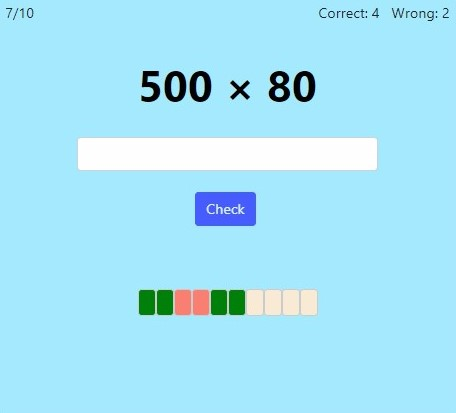MM Practice
×
Multiplication
Division
Place Value
Fractions & Decimals
Measurement
Statistics & Probability
Pre-Algebra
Money
Kindergarten

# Multiply Whole Numbers With ZerosOnline practice for grades 4-6

On this page, you can practice multiplying numbers with zeros, such as 20 × 300 and 7,000 × 200, using mental math. Students usually learn this topic in 4th grade, but 5th and 6th grade students usually benefit from practicing it too.

The principle for solving such multiplication problems is this: multiply the numbers without the zeros first. Then, tag as many zeros as there are in the factors to the end of the product.

For example, to solve 20 × 300, first multiply 2 × 3 = 6. Then, since 20 has one zero and 300 has two, tag three zeros to the end of 6, to get 6,000.

You can also practice the opposite operation, division of numbers ending in zeros here.

You can choose the level of difficulty, timed or untimed practice, and the number of practice problems.

Screenshot:OR
Practice for a set time: min

Allow my comment to be posted on this site